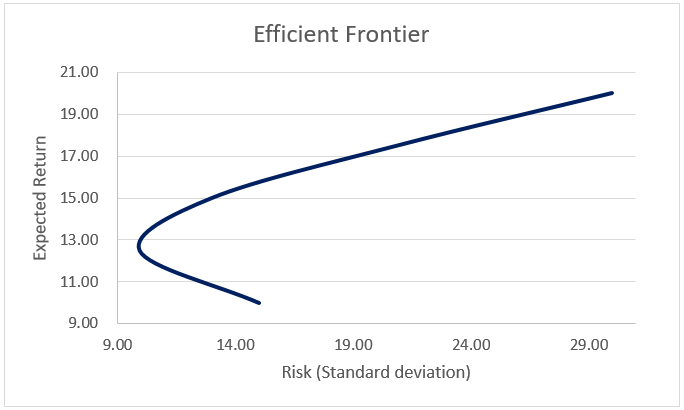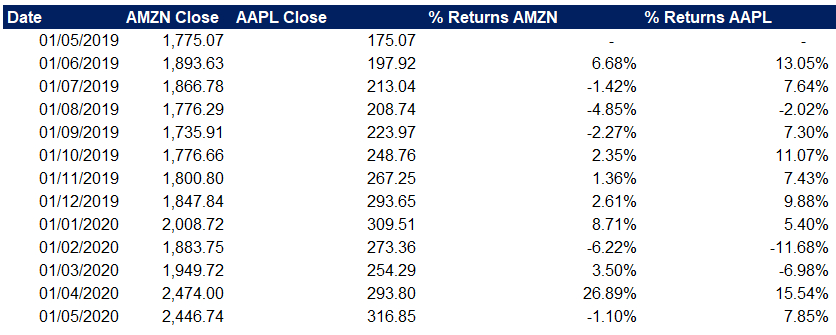## Optimal Portfolios and the Efficient Frontier

There’s a widespread assumption in investing that more risk equals increased potential returns. The theory behind the Efficient Frontier and Optimal Portfolios states that there’s an optimal combination of risk and return.

The theory relies on the assumption that investors prefer portfolios that generate the most substantial possible return with the least amount of involved risk. We refer to these as optimal portfolios, and they form the efficient frontier curve.

## Optimal Portfolio

An optimal portfolio is one that occupies the ‘efficient’ parts of the risk-return premium spectrum. It satisfies the requirement that no other collection exists with a higher expected return at the same standard deviation of the return (risk measure).

Different combinations of assets produce different levels of return. The optimal portfolio concept represents the best of these combinations, those that provide the maximum possible expected return for a given level of acceptable risk.

The relationship between assets is an essential part of the optimal portfolio theory. Some prices move in the same direction under similar circumstances, while others go in opposite directions. The more out of sync these price developments are, the lower the covariance between two assets is, which translates into lower overall risk.

The optimal portfolio does not focus on investments with either high expected returns or low risk. It aims to balance stocks carrying the best potential returns with acceptable risk. When we plot these, we get the Efficient Frontier.

## The Efficient FrontierThe Efficient Frontier concept has its roots in the 1950s, and it’s a pillar of Modern Portfolio Theory.

The Efficient Frontier is a set of optimal portfolios that give the highest possible expected return for a given risk level or the lowest risk for a desired expected return. Portfolios below the efficient frontier are sub-optimal, as they don’t provide enough returns for their risk levels.

Returns depend on the investments combined in the portfolio. Those on the right of the efficient frontier have higher risk levels for the defined rate of return. Risk seeking investors would look at these portfolios, while risk-averse investors would look on those on the left side.

For this model, we consider the standard deviation of the return on the asset as its risk measure. Lower covariance between portfolio securities results in smaller portfolio standard deviation. Therefore, optimal portfolios that comprise the efficient frontier tend to have a higher degree of diversification. The compounded annual growth rate is a common choice for the return component, while the annualized standard deviation represents the related risk.

It’s a graphical way to illustrate the portfolios that maximize the return for the assumed risk. Profitability depends on the combination of held investments. Ideally, we would want to create a collection of assets with a high yield and aggregated standard deviation (risk level), which is lower than the individual assets’ standard deviation.

Lower synchronization rates between the investments (lower covariance) mean lower standard deviation and risk. If such optimization of return versus risk is successful, the portfolio will lie on the efficient frontier curve. Optimal portfolios on the efficient frontier tend to be more diversified.

The curve is essential in showing how diversification improves the risk/reward profile for the investor. It shows that the relation between risk and return is non-linear. There is a diminishing marginal return to risk; adding more risk does not gain an equal return. Instead, each additional unit of risk adds a smaller and smaller amount of return to the portfolio.

## Assumptions and Limitations

The theory behind the Efficient Frontier relies heavily on some assumptions, not all of which represent reality. The underlying assumptions for the optimal portfolio are focused primarily on the investors:

• We expect investors to be rational and all have access to the same information;
• They are all risk-averse and share the goal to maximize returns;
• No single investor can influence the market;
• All market players have access to unlimited funds at a risk-free rate;
• We assume that asset returns follow a normal distribution;
• Investors base all decisions on the market on expected returns and standard deviation as a measure of risk.

## Plotting the Efficient Frontier

To form the curve of the Efficient Frontier, we need to keep three main factors in consideration:

• Expected return of portfolios;
• Variance or standard deviation as a measure of the return variability (risk);
• The covariance of the assets in the portfolio.

For a two-asset portfolio, we can calculate the expected return as:Where:

• Er(P/A/B) is the expected returns of the portfolio, asset A, and asset B;
• wA/B are the weights of assets A and B in the portfolio.

To calculate the standard deviation as the risk measure, we use the following formula:Where:

• SDP/A/B is the standard deviation (risk measure) of the portfolio, asset A, and asset B;
• cor(A,B) is the correlation coefficient between the returns of assets A and B.

We then plot expected returns on the y-axis and the standard deviation as a risk-measure on the x-axis. It shows the risk-return trade-off of portfolios.

## Example Efficient Frontier Determination

We will look at an example with two publically traded companies, Apple (AAPL) and Amazon (AMZN). We can download the ticker data for Amazon and Apple from Yahoo Finance. We take the monthly Close prices for the past year and calculate the return % on a month-to-month basis.With this, we can now calculate our Mean, Variance, Standard Deviation, and Correlation, using the respective Excel formulas AVERAGE, VAR.P, STDEV.P, and CORREL.

Then we can apply the formulas outlined above to calculate the portfolio return and risk (standard deviation) of a 50%-50% split.However, to find our optimal portfolio, let us look at various weight combinations between the two assets. For all possible collections with a 10% step, we calculate the expected return and standard deviation as a risk measure.The formula for Portfolio return in Excel will use the means we calculated earlier and the respective weight split for each portfolio.To calculate our risk measure, we apply the standard deviation formula, as outlined above:That way, we have our Minimum Variance Frontier data ready to plot. By looking at the portfolio with the minimum risk, we can separate the collections that have the same risk level but provide lower expected returns. This is how we separate the Efficient Frontier.With these helper calculations on the right, we can now go ahead and plot the data on a Scatter Diagram.From our calculations above, we note that we achieve the lowest risk level within our portfolio variants at a 40%-60% split. However, we only analyzed one year. If we head over to Portfolio Visualizer and run their Efficient Frontier tool for Apple and Amazon, for all available ticker data since 1997, we get the following tangent portfolio.We can conclude that if our portfolio consists only of Amazon and Apple shares, we need to keep the split around 40%-60%, to achieve the maximum expected return with the lowest risk level.

## Conclusion

The Efficient Frontier helps us identify and visualize a combination of assets with the optimal level of expected return for any given risk level. Portfolios on the curve are most efficient. Other collections either have lower expected returns for the same risk level or introduce higher risk levels for the same expected returns.

By plotting the Efficient Frontier, we can perform a more thorough analysis of our investment opportunities and make more informed decisions.

Show your support by sharing this article with colleagues and friends via the social icons on the side.

## And Get a FREE Benchmark Analysis Template## FCCA, FMVA

Hi! I am a finance professional with 10+ years of experience in audit, controlling, reporting, financial analysis and modeling. I am excited to delve deep into specifics of various industries, where I can identify the best solutions for clients I work with.

In my spare time, I am into skiing, hiking and running. I am also active on Instagram and YouTube, where I try different ways to express my creative side.

### 5 Responses

1. nath says:

why you didn take close adjusted price?

1. Dobromir Dikov says:

Hi Nath, to be honest, I am not sure which one is better, being that close price is the actual ‘cash’ price, whereas adjusted price includes dividends, etc.
I have seen models use both but never thought much about it. Do you have any backing as to why the adjusted close price is better? Please share if you do.

2. Eric says:

Thank you for the form!! I use the net price that includes reinvested dividends, etc. so that I can calculate full returns. I had a question though on why you use the arithmetic returns instead of the geometric returns. I thought you had to use geometric returns to get an accurate Compound Annual Growth Rate. Can you advise? I am confused on this point.

1. Dobromir Dikov says:

Hi Eric, thank you for your comment!
I am not sure I fully understand what you mean by your question. As we want to get the average return that the different assets can generate, we use the mean, because a cagr would compound returns, which is not what we want as I understand it. Please let me know if I misread your question!
Regards,
Dobri

3. Claudia says:

Can you perform this analysis on crypto assets, like bitcoin?